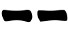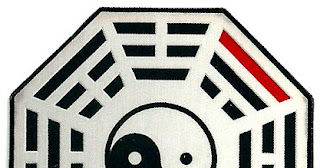## Sunday, 10 July 2016

Book pages can be very helpful to cast I Ching hexagrams. Russell Cottrell devised a simple method that uses even book's pages. Directly from his post on Clarity:
1. Fan the pages of a book (such as the I Ching).
2. Very casually divide the pages at a random position, as if cutting a deck of cards.
3. Look at the last two digits of the number of the even-numbered page (generally on the left).
4. If it is divisible by four, count it as “tails” or 2.
5. If not, count it as “heads” or 3.
6. To make the math easier, subtract a multiple of 4 from the page number, such as 40 or 80.
7. Three divisions determine one line, just like using coins.
[...] The edges of the pages must be fairly clean and smooth, without irregularities that could throw off the results.

#### Probabilities

As Russel suggested, the probabilities are the same as the three coins method. In fact, picking at random an even number, the chance of it being divisible by 4 is 1/2 and hence:
Prob(6) = Prob(9) = 1/8 = 12.5%
Prob(8) = Prob(7) = 3/8 = 37.5%
Prob(yin) = Prob(yang) = 1/2

## Sunday, 3 July 2016

This method was devised by Jason A. Wolcott and recorded in the I Ching methods page on the Lucky Mojo site.
It uses two dices: one with 20 faces (1d20) and one with eight faces (1d8) which are very easy to find being commonly used for bard games.
It proceeds as follows:
1. Roll the dice;
2. Draw the line:
• If the 20 sided die shows an even number (2,4,...,20), draw;
• If the 8 sided die shows 1, it's a moving line
• If the 20 sided die shows an odd number (1,3,...,19), draw;
•  If the 8 sided die shows 6, 7 or 8, it's a moving line
3. Repeat steps 1-2 other five times drawing the hexagram from the bottom to the top

#### Probabilities

The 20 sided die gives the nature of the line (yin or yang) with probability 1/2. The process is designed to give the same probability of the Method of 16 one:
Prob(6) = 1/2 * 1/8 = 1/16
Prob(8) = 1/2 * 7/8 = 7/16
Prob(7) = 1/2 * 5/8 = 5/16
Prob(9) = 1/2 * 3/8 = 3/16
Prob(yin) = Prob(yang) = 1/2

#### Variations

Since the the 20 sided die is only used for even/odd probability it can be replaced by any die with an even number of faces.

## Saturday, 2 July 2016

### Improved Yarrow Stalk Divination

by Joel Benson

The traditional forty-nine-stalk method of divination has long been known and has been supplanted by the quicker three-coin method and other relatively rapid modern methods, for example involving special dice or sixteen ritual objects such as colored marbles.
I found it odd that in all the literature I examined, the assumption seems to be that yarrow divination is necessarily limited to the ancient method of sorting, counting and adding random bundles of thin sticks. I decided there has to be an easier way to perform a Yi Jing divination with yarrow, while enhancing the wonderful tactile feel and spiritual presence of yarrow stalks.
After some thought I realized there is a simpler, quicker way to manipulate a limited number of sturdy, relatively thick yarrow stalks to randomly define primary hexagrams using the established Yi Jing yarrow probabilities.
In my improved divination method only eight yarrow stalks are required. I prefer stalks about 10 inches long and about .25 inches in diameter. Each of the stalks has yin and yang ends that are marked in any way that may be preferred to designate old or young yin/yang in the accepted yarrow divination proportions.
My preferred way of marking the eight yang ends of the stalks is to insert a magnetized pin in each yang end. So the yang ends generate magnetic energy in accordance with their yang nature. The yin ends have no such pins and are therefore identified by their receptive, non-energy nature. A black end color is used to designate a single old yin end and three old yang ends, as required for yarrow divination. The remaining ends that are not blackened define seven young yin and five young yang ends.
In the divination ritual, the eight stalks are shuffled and then an end of one stalk is selected at random to designate the first level of the primary hexagram. The selected stalk is then returned to the group of eight, the stalks are shuffled again and a random end of a stalk is selected to define the second level of the primary hexagram. This is continued until the six-line hexagram is defined.
This method is similar to Rule of 16 divinations, except colors need not be memorized and only eight sturdy yarrow stalks are used. Also, the magnetic yang ends may be detected by a compass to add a mystical touch to the divination.
So a few substantial yarrow stalks may be used in an easy, quick and modern style of divination. Following is an image of eight yarrow stalks of the type described and an image of a magnetic pin inserted at the yang end of a stalk to illustrate how the yang ends of the stalks are marked.In the picture you can see a simple device to cast hexagrams: an octagonal tablet (around 5cm wide) with one face displaying the eight trigram in the Fuxi Earlier Heaven arrangement and the other side with trigrams in the King Wen Later Heaven arrangement. Four lines, two on each side, are painted so that three yang (solid) lines and one yin (broken) lines are red.
To cast a hexagram the process is as follows:
1. Turn and flip the tablet without looking;
2. Stop and put a finger on one of the sides;
3. Draw the topmost line on that side. If it's red, it's a moving line;
4. Repeat steps 1-3 other five times drawing the hexgram from bottom to top
I built the one you see in the picture with laminated cardboard. You can see it is a little bit damaged in certain points due to the overuse (it has been my preferred method for more than a year). I believe it would be great if casted (or 3d printed) in metal.

#### Probabilities

Considering the two faces, the eight sides and the way the red lines are painted, the probabilities are the same as the Method of 16:

Prob(6) = 1/16
Prob(8) = 7/16
Prob(7) = 5/16
Prob(9) = 3/16
Prob(yin) = Prob(yang) = 1/2

This method was devised and published on the internet by i@no-self in 2001. I took the liberty of changing the procedure a little to make it easier to memorize.

In this method, an hexagram is selected at random and the line is derived on the basis of the upper and lower trigrams:

1. Open an I Ching book to a random page and look at the hexagram that page relates to;
2. If the lower trigram has one or three yin (broken) lines, you got a yin line:
• if the upper trigram has three broken line, draw;
• otherwise  draw;
3. If the lower trigram has one or three yang (solid) lines, you got a yang line:
• if the upper trigram has exactly one solid line, draw;
• otherwise draw;
4. Repeat steps 1-2 other five times drawing the hexagram from bottom to top.
If you find difficult to memorize the rules, you can use the table below which is structured according the original formulation by i@no-self.

#### Probabilities

The actual probabilities of each line depends on how the book has been typeset (some hexagram may have more pages than others) and by how much one is inclined to pick up the pages that are towards the end or the beginning of the book.
Assuming, everything considered, that any combination of upper/lower trigrams has the same probability to be selected, the probabilities for each line are the same as the Method of 16:

Prob(6) = 1/16
Prob(8) = 7/16
Prob(7) = 5/16
Prob(9) = 3/16
Prob(yin) = Prob(yang) = 1/2

## Friday, 1 July 2016

Anoher method using a single dice:
1. Roll a die;
2. Draw the line:
• If it's an even number (2,4,6), draw;
• If it's an odd number (1,3,5), draw;
3. If it's 1 or 6, it's a moving line;
4. Repeat steps 1-3 other five times drawing the hexagram from the bottom to the top.

#### Probabilities

The probabilities are similar to the three coins one but there are more chances of producing moving lines:

Prob(6) = Prob(9) = 1/6 = 16.7%
Prob(8) = Prob(7) = 2/6 = 33.3%
Prob(yin) = Prob(yang) = 1/2

#### Variations

I've found that the company Antony Publishing sells dice that works with the same principle but can directly provide the resulting line (moving lines are marked with a dot). These two images have been taken directly from their site:Single dieSet of six dice